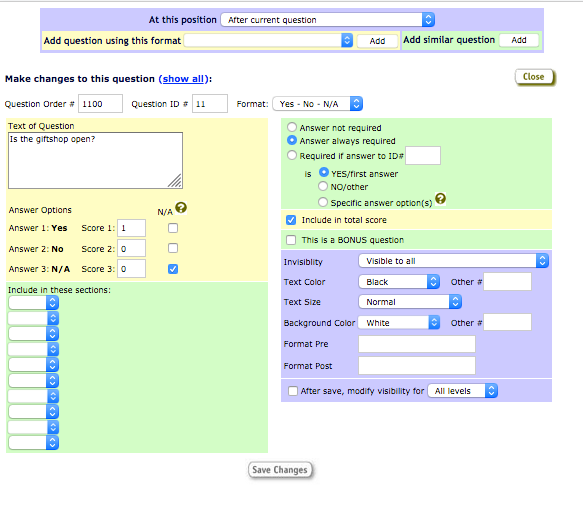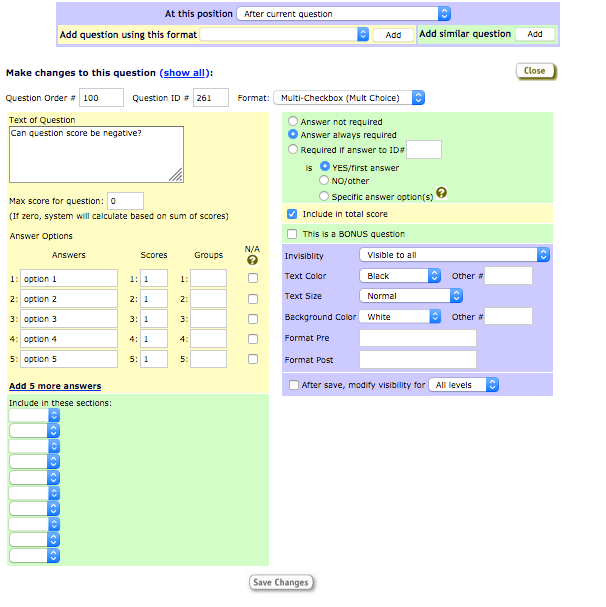Most of Sassie and Dragon is built for surveys that are scored in some way. Here are some terms you'll come across when setting up surveys and looking at results in reporting.

Job Score: an individual job (shop) in Sassie has a score, which is the points earned on the shop, divided by the possible points on the shop.  A Job Score is a percentage.

Sectional Score: Each section on a shop can have a score, also calculated as earned points divided by possible points.

Possible Points: The highest point value of an answer option in the question determines the "possible points".

Bonus Question: A bonus question has a point value for one or more of its answer options, but no Possible points, so it only adds points to the shop's earned points, never to the possible points.

NA: an answer option within a question can be marked as "NA". You have the option, in the Survey settings, to have this answer option add no possible points to the shop's possible points when selected.

Examples:

A yes/no question can have a point assigned to the "yes" answer option, and typically zero (0) points assigned to the "no" answer option. When Yes is selected, the question scores 1/1, or one earned point out of one possible point. When No is selected, the question scores 0/1 or 0 earned points and 1 possible point. The "yes" answer option can be assigned any point value you wish.A yes/no/NA question can be similar to yes/no but with a third, Not Applicable answer option, which has no point value but will not affect the shop's score negatively either. When answered this NA option, the question can score 0/0, zero earned points and zero possible points, if the survey is set up this way.A multi-check question has multiple answer options that can be selected at the same time. If each answer option of 5 options is assigned 1 point, and 3 are selected, the question would score 3/5, three earned points, 5 possible points.